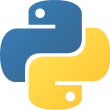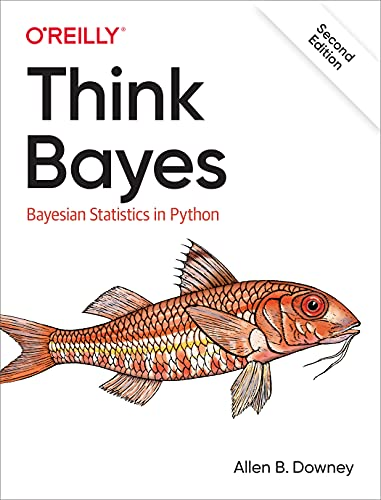Language
• Python 3Reading time
• Approximately 33 daysWhat you will learn
• Numerical Programming and Data MiningAuthor
• Allen B. DowneyPublished
• 2 years, 6 months agoPackages you will be introduced to
• pymc3If you know how to program, you're ready to tackle Bayesian statistics. With this book, you'll learn how to solve statistical problems with Python code instead of mathematical formulas, using discrete probability distributions rather than continuous mathematics. Once you get the math out of the way, the Bayesian fundamentals will become clearer and you'll begin to apply these techniques to real-world problems.

Bayesian statistical methods are becoming more common and more important, but there aren't many resources available to help beginners. Based on undergraduate classes taught by author Allen B. Downey, this book's computational approach helps you get a solid start.

• Use your programming skills to learn and understand Bayesian statistics
• Work with problems involving estimation, prediction, decision analysis, evidence, and Bayesian hypothesis testing
• Get started with simple examples, using coins, dice, and a bowl of cookies
• Learn computational methods for solving real-world problems
The author Allen B. Downey has the following credentials.

• Professor at Franklin W. Olin College of Engineering
• Professor at Harvard University, one of the best universities in the world